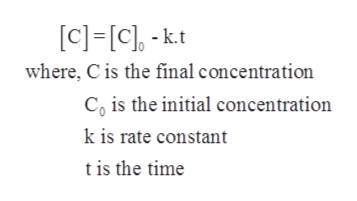For the zeroth-order reaction: C → products, -Δ[C]/Δt = k, which of the following graphs would be expected to give a straight line?[C]2 vs. t[C] vs. t1/[C] vs. tln[C] vs. t

Question

For the zeroth-order reaction: C → products, -Δ[C]/Δt = k, which of the following graphs would be expected to give a straight line?

 [C]2 vs. t [C] vs. t 1/[C] vs. t ln[C] vs. t
Step 1

Rate of reaction represents the change of concentration of a reactant or a product with respect to time. It can be expressed either by reduce amount of reactant in per unit time or increase amount of product in per unit time.

Step 2

The reaction in which rate of reaction is constant and is independent of the concentration of reacting species that is reactants is known as zero order reaction.

Step 3

The given reaction is C → Products

Therefore, the integrated rate ...help_outlineImage Transcriptionclose[c] [c]-k.t where, C is the final concentration Co is the initial concentration k is rate constant tis the time fullscreen

Want to see the full answer?

See Solution

Want to see this answer and more?

Our solutions are written by experts, many with advanced degrees, and available 24/7

See Solution
Tagged in

Chemical Kinetics